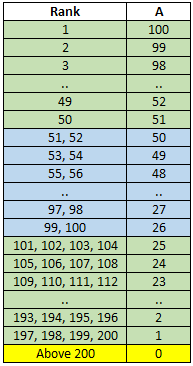Nurimisaki Nomad ( 17th Sep to 7th Oct )

#### ?

O Player Country Solve Time Pen E Finish Time Clicks Replay

#### ?

A B C
A is determined by player's rank on that day. The table below demostrates the link between rank and A.B is puzzle difficulty indicator.
Players solving all 16 puzzles will always get 1600 points as B.
B for each puzzle will be calculated based on the following formula after the official time for the puzzle is over.
B for Nth puzzle = 1600 * (Avg of Top 10 players's time of Nth puzzle) / [ Sum of (Avg of Top 10 players's time of all puzzles) ]
First 100 players to submit the puzzle will get C as 100 to 1
Final Leaderboard considers cumulative of A + B for each day. Final Leaderboard considers cumulative of C for each day.

# N Player A + B

# N Player C

#### Player

Puzzle A B C

N = Number of puzzles solved within Official Period / Number of puzzles solved
A = SUM(Top 100 players get points from 100 to 1 based on their ranking for that day)
B = SUM(Puzzle Points calculated based on average solving time of top 10 players)
C = SUM(First 100 players get points from 100 to 1 based on their solving end time for that day)